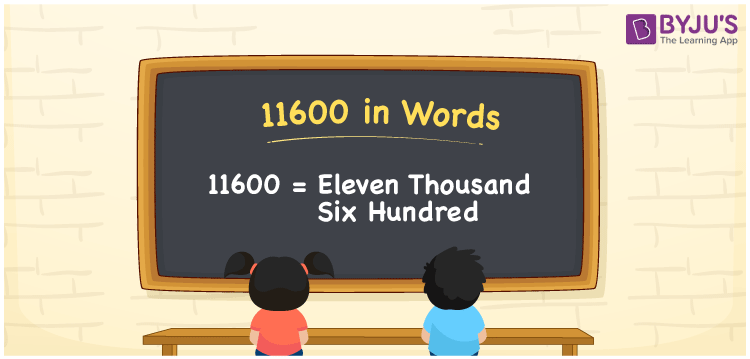# 11600 in Words

11600 in words can be written as Eleven Thousand Six Hundred. Students will be able to learn the conversion of 11600 in words which will help them understand the applications of numbers in our daily lives. If you buy a gadget for Rs. 11600, then you can say that “I have bought a gadget for Eleven Thousand Six Hundred Rupees”. The  number 11600 can be written in words using the English alphabet. The numbers in words can be grasped easily by the students using the resources given at BYJU’S. 11600 in English can be read as “Eleven Thousand Six Hundred”.

 11600 in words Eleven Thousand Six Hundred Eleven Thousand Six Hundred in Numbers 11600

## 11600 in English Words## How to Write 11600 in Words?

Students will learn about the conversion of 11600 into words from place value charts. The number 11600 has four digits. For 11600, the place value chart is prepared in a table form to help students understand it effectively.

 Ten thousand Thousands Hundreds Tens Ones 1 1 6 0 0

11600 in expanded form is explained in brief here:

1 × Ten Thousand + 1 × Thousand + 6 × Hundred + 0 × Ten + 0 × One

= 1 × 10000 + 1 × 1000  + 6 × 100 + 0 × 10 + 0 × 1

= 10000 + 1000 + 600

= 11600

= Eleven Thousand Six Hundred

Therefore, 11600 in words is written as Eleven Thousand Six Hundred.

11600 is a natural number that precedes 11601 and succeeds 11599.

11600 in words – Eleven Thousand Six Hundred

Is 11600 an odd number? – No

Is 11600 an even number? – Yes

Is 11600 a perfect square number? – No

Is 11600 a perfect cube number? – No

Is 11600 a prime number? – No

Is 11600 a composite number? – Yes

## Frequently Asked Questions on 11600 in Words

### How do you write 11600 in words?

11600 can be written as “Eleven Thousand Six Hundred” in words.

### Is 11600 an even number?

11600 is an even number because it is divisible by 2.
11600/2 = 5800

### How can Eleven Thousand Six Hundred be written in numbers?

Eleven Thousand Six Hundred can be written in numbers as 11600.